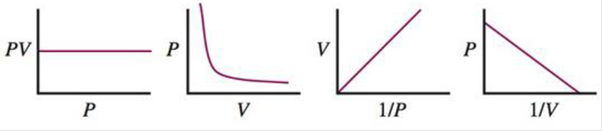# Boyle’s law can be represented graphically in several ways. Which of the following plots does not correctly represent Boyle’s law (assuming constant T and n )? Explain.### Chemistry: An Atoms First Approach

2nd Edition
Steven S. Zumdahl + 1 other
Publisher: Cengage Learning
ISBN: 9781305079243

#### Solutions

Chapter
Section### Chemistry: An Atoms First Approach

2nd Edition
Steven S. Zumdahl + 1 other
Publisher: Cengage Learning
ISBN: 9781305079243
Chapter 8, Problem 23Q
Textbook Problem
1009 views

## Boyle’s law can be represented graphically in several ways. Which of the following plots does not correctly represent Boyle’s law (assuming constant T and n)? Explain.Interpretation Introduction

Interpretation:  From the given plots, a plot that doesn’t correctly represent Boyle’s law should be identified.

Concept introduction:

Boyle’s law can defined as,

At constant temperature and number of moles, volume of a gaseous container is inversely

proportional to the pressure excreted on the container.

Mathematically,

P1V

Or

PV=K

Where,

P = pressure in atmospheres

V= volumes in liters

K= a constant for a particular gas at given temperature

By comparing two systems at different conditions

P1V1=P2V2

### Explanation of Solution

The plot that doesn’t correctly represent Boyle’s law

According to Boyle’s law, at constant temperature and number of moles, volume of a gaseous container is inversely proportional to the pressure excreted on the container.

That is ‘P’ and ‘V’ is inversely proportional.

That is,

P1V , by changing the proportionality sign

PV=K

By rearranging the equation,  P=k(1/V)

This is in the form of the straight line equation y=mx+b

Here in the given plot x - axis 1/V and y – axis is pressure. On accordance with Boyle’s law The P versus 1/V plot should be a linear with positive slope and y-intercept of zero.

Here the given plot P versus 1/V is a plot with negative slope. A negative slope implies that y –axis always decreases when x-axis increases and y- axis always increases when x-axis decreases.

So, the given plot P versus 1/V doesn’t correctly represent Boyle’s law.

The plots that correctly represent Boyle’s law

a)

b)

### Still sussing out bartleby?

Check out a sample textbook solution.

See a sample solution

#### The Solution to Your Study Problems

Bartleby provides explanations to thousands of textbook problems written by our experts, many with advanced degrees!

Get Started

Find more solutions based on key concepts
A B vitamin often forms part of an enzyme's active site, where a chemical reaction takes place. T F

Nutrition: Concepts and Controversies - Standalone book (MindTap Course List)

Provide two reasons why meiosis leads to genetic variation in diploid organisms.

Human Heredity: Principles and Issues (MindTap Course List)

How is waters density affected by freezing? Why does ice float?

Oceanography: An Invitation To Marine Science, Loose-leaf Versin

Assuming the cost of energy from the electric company is 0.110/kWh, compute the cost per day of operating a lam...

Physics for Scientists and Engineers, Technology Update (No access codes included)